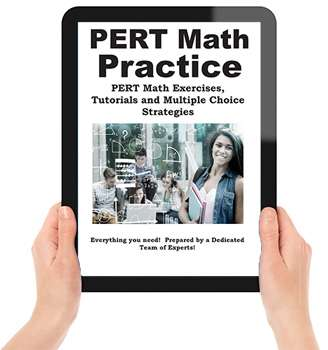## The PERT math test contains 50 questions, all multiple choice, in the following areas:

### Basic Math

Fractions, Decimals and Percent
Order of Operations
Word Problems

### Basic Algebra

Linear equations with 1 and 2 variables
Binomials and monomials
Solve Inequalities

### Basic Algebra Practice

Basic Algebra
Linear Equations (1 variable)
Linear Equations (2 variables)
Operations with Polynomials
Solving Inequalities – Quick tutorial and practice questions

### Basic Geometry

Area, Volume and Perimeter
Slope of a line
Identify linear equations from a graph
Calculate perimeter, circumference and volume
Solve problems using the Pythagorean theorem

## Need to brush up on Your math? This is the book for you!

### Here is what the PERT Math Workbook can do for you

• Learn then practice your math skills! Practice test questions are the best way to prepare for an exam and this is the book that you need to fully prepare for the PERT math test.
• Practice Tests familiarize you with the exam format and types of questions, giving you more confidence when you take the exam.
• Practice tests are a critical self-assessment tool that reveals your strengths and weaknesses.
• Practice tests allow you to practice your exam time management – a critical exam-writing skill that can easily improve your grade substantially.
• Practice tests reduce Test Anxiety, one of the main reasons for low marks on an exam. Hundreds of questions with detailed solutions and explanations to improve your understand of the basic concepts behind the questions.
• Learn powerful multiple choice strategies designed by exam experts! Includes tips and multiple choice strategies to increase your score you won’t find anywhere else!

Practice Really Does Make Perfect!

The more questions you see, the more likely you are to pass the test. And between our study guide and practice tests, you’ll have over 300 practice questions that cover every category.

Our practice test questions have been developed by our dedicated team of experts. All the material in the study guide, including every practice question, are designed to engage your critical thinking skills needed to pass the test!

Heard it all before?

Maybe you have heard this kind of thing before, and don’t feel you need it. Maybe you are not sure if you are going to buy this book.

Remember though, it only a few percentage points divide the PASS from the FAIL students!

Even if our test tips increase your score by a few percentage points, isn’t that worth it?

### Postsecondary Education Readiness Test PERT Math Practice WorkbookPERT Math Workbook

Includes over 200 math practice questions, How to study for a math test, math tips, tricks and shortcuts, multiple choice strategies and more! Prepared by a dedicated team of experts!

ORDER NOW

Language: English

Price:
APP: USD\$14.99
Condition: New
Availability: In Stock

Getting Started
Making a Study Schedule 10

Basic Math
Fraction Tips, Tricks and Shortcuts 16
Converting Fractions to Decimals 18
Converting Fractions to Percent 19
Decimal Tips, Tricks and Shortcuts 22
Converting Decimals to Fractions 22
Converting Decimals to Percent 22
Percent Tips, Tricks and Shortcuts 23
Converting Percents to Decimals 24
Converting Percents to Fractions 25
Exponents: Tips, Shortcuts 26
Order of Operation 28
Basic Math Practice 31

How to Solve Word Problems
Types of Word Problems 56
Word Problem Practice Questions 70

Basic Geometry
Pythagorean Geometry 95
Geometry Practice 102

Algebra
Solving One-Variable Linear Equations 125
Factoring Polynomials 126
Solving Two-Variable Linear Equations 129
Simplifying Polynomials 131
Factoring Polynomials 131
Solving Inequalities 134
Algebra Practice 138

Basic Math Multiple Choice
Strategy and Shortcuts 177

How to Study for a Math Test

How to Prepare for a Test
The Strategy of Studying 185